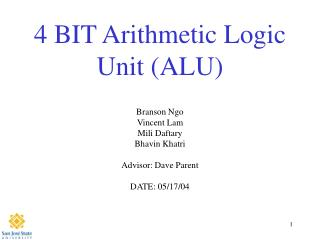Download Presentation4 BIT Arithmetic Logic Unit (ALU)# 4 BIT Arithmetic Logic Unit (ALU) - PowerPoint PPT Presentation

Download Presentation##### 4 BIT Arithmetic Logic Unit (ALU)

Download Policy: Content on the Website is provided to you AS IS for your information and personal use and may not be sold / licensed / shared on other websites without getting consent from its author. While downloading, if for some reason you are not able to download a presentation, the publisher may have deleted the file from their server.

- - - - - - - - - - - - - - - - - - - - - - - - - - - E N D - - - - - - - - - - - - - - - - - - - - - - - - - - -
##### Presentation Transcript

1. 4 BIT Arithmetic Logic Unit (ALU) Branson Ngo Vincent Lam Mili Daftary Bhavin Khatri Advisor: Dave Parent DATE: 05/17/04

2. Agenda • Abstract • Introduction - Why - Background Information • Project Summary • Project Details - schematic - Layout - LVS report • Longest Path Calculations • Lessons Learned • Summary • Acknowledgements

3. Abstract • Our group has designed a 4 Bit Arithmetic Logic Unit (ALU) that can perform the following functions: - NAND - NOR - XOR - Full Adder The area of our design is 1.04 x 10^-7 m2 Power = I*V = (0.012A)*(2.5V) = 30mW

4. Introduction • An ALU is the fundamental unit of any computing system. • Understanding how an ALU is designed and how it works is essential to building any advanced logic circuits. • Using this knowledge and experience, we can move on to designing more complex integrated circuits.

5. Introduction – contd. • We have designed an ALU to handle two inputs of 4 bits each to produce a required output based on the output selector line. • The possible outputs are Nand, Nor, Xor and Sum as shown in the table.

6. Project Summary • The design uses the concept of parallel computing. • All the different logical functional units like Nand, Nor, etc. are cascaded together and produce outputs in parallel at the Mux input. • The benefit of this design is that all the computations are done in parallel and available simultaneously, so no clock resources are wasted. The Mux is then simply used to select the required output.

7. Project Details • There are total of 19 pin outs in our design including vdd and gnd. • There are 9 D Flip-flops at the input and 4 at the output. • There are 4 4x2 Mux’s to select the output

8. Project Details – contd. • Create Schematics and layouts for Nand, Nor, Xor, Adder, flip-flop, and Mux in the Cadence tool. • Test the schematics using test bench. • Create Schematic and layout for 1 bit ALU now using the schematics for the basic logical units. • Test the schematic for 1 bit ALU. • Create the schematic for 4 bit ALU. • Cascade the single bit ALU layouts to create a 4 bit ALU layout. • Run the DRC, extracted and LVS check to verify the design.

9. Longest Path Calculations

10. 4 Bit ALU Schematic

11. Layout

12. Verification- LVS Check

13. Simulation

14. Simulation- Contd

15. Lessons Learned • Don’t route in Poly • Learned to fix the LVS Error • Learn how to use Cadence tool • Learn how to design an integrated circuit • Make design decisions to create an efficient design.

16. Summary • The project taught us how to design a basic integrated circuit. This is a stepping stone for more complex circuits. • Our project has 321 transistors and 19 terminals. • The area of our design is = 1.04 x 10^-7m2 • The power is 30mW.

17. Acknowledgements • Thanks to professor David Parent for helping us out at all the points where we were stuck. • Thanks to Cadence Design Systems for VLSI Lab. • Thanks to our colleagues in the lab.